Скачать презентацию 12 0 Chapter 12 Mc Graw-Hill Irwin Cost of

c221c13400ec2e32d41034e443e3b337.ppt

• Количество слайдов: 42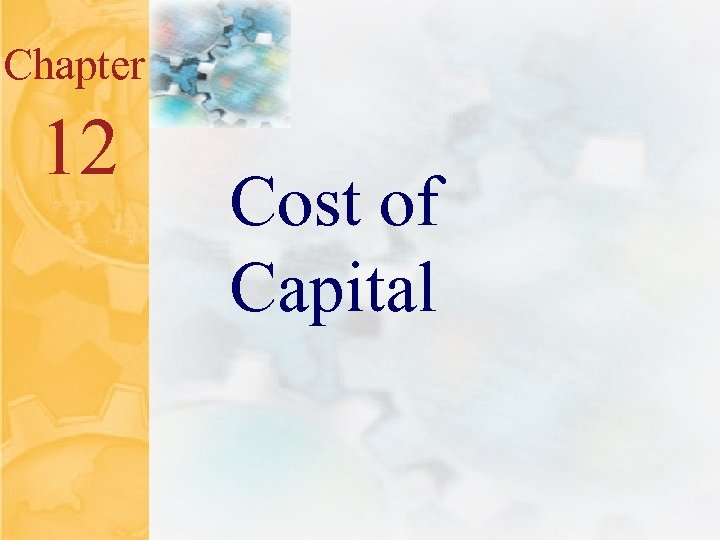12. 0 Chapter 12 Mc. Graw-Hill/Irwin Cost of Capital © 2001 The Mc. Graw-Hill Companies All Rights Reserved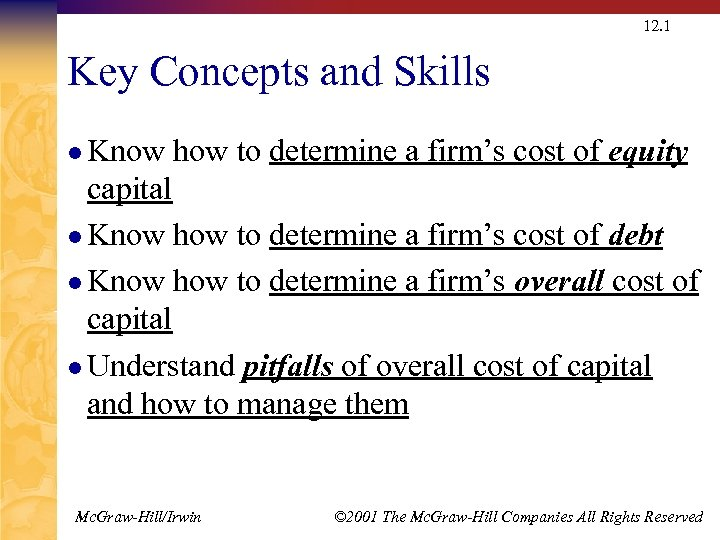12. 1 Key Concepts and Skills l Know how to determine a firm’s cost of equity capital l Know how to determine a firm’s cost of debt l Know how to determine a firm’s overall cost of capital l Understand pitfalls of overall cost of capital and how to manage them Mc. Graw-Hill/Irwin © 2001 The Mc. Graw-Hill Companies All Rights Reserved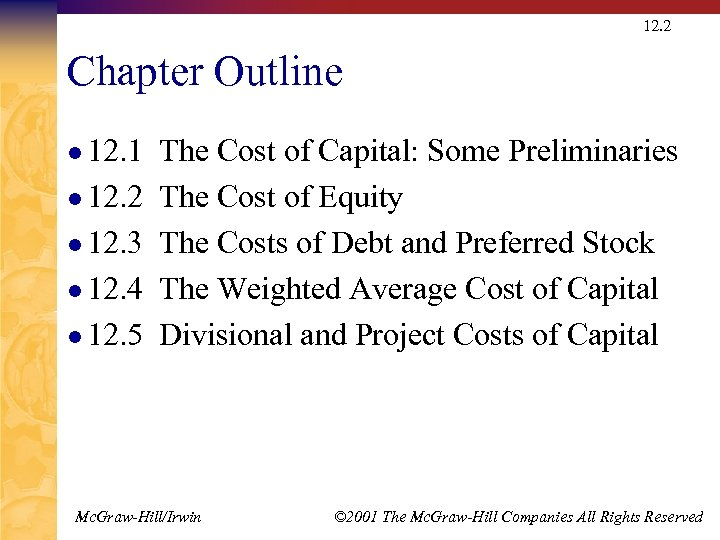12. 2 Chapter Outline l 12. 1 l 12. 2 l 12. 3 l 12. 4 l 12. 5 The Cost of Capital: Some Preliminaries The Cost of Equity The Costs of Debt and Preferred Stock The Weighted Average Cost of Capital Divisional and Project Costs of Capital Mc. Graw-Hill/Irwin © 2001 The Mc. Graw-Hill Companies All Rights Reserved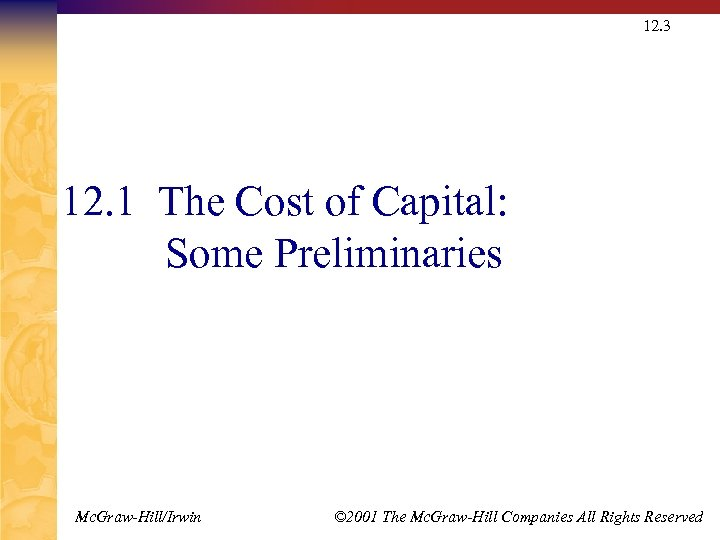12. 3 12. 1 The Cost of Capital: Some Preliminaries Mc. Graw-Hill/Irwin © 2001 The Mc. Graw-Hill Companies All Rights Reserved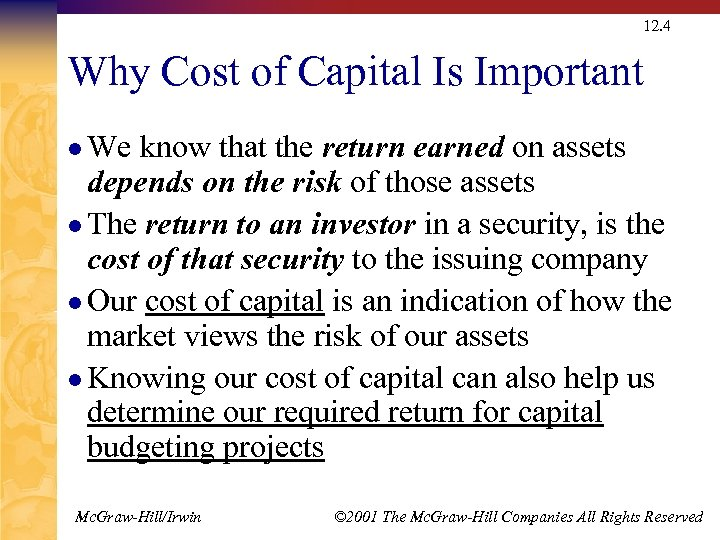12. 4 Why Cost of Capital Is Important l We know that the return earned on assets depends on the risk of those assets l The return to an investor in a security, is the cost of that security to the issuing company l Our cost of capital is an indication of how the market views the risk of our assets l Knowing our cost of capital can also help us determine our required return for capital budgeting projects Mc. Graw-Hill/Irwin © 2001 The Mc. Graw-Hill Companies All Rights Reserved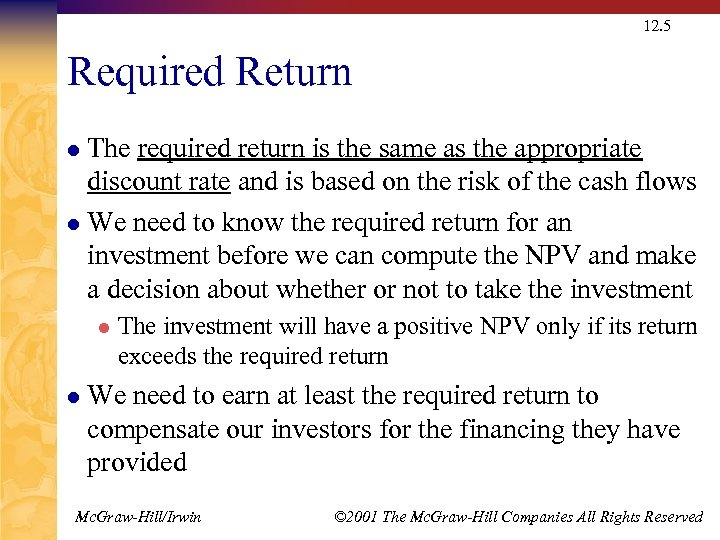12. 5 Required Return The required return is the same as the appropriate discount rate and is based on the risk of the cash flows l We need to know the required return for an investment before we can compute the NPV and make a decision about whether or not to take the investment l l l The investment will have a positive NPV only if its return exceeds the required return We need to earn at least the required return to compensate our investors for the financing they have provided Mc. Graw-Hill/Irwin © 2001 The Mc. Graw-Hill Companies All Rights Reserved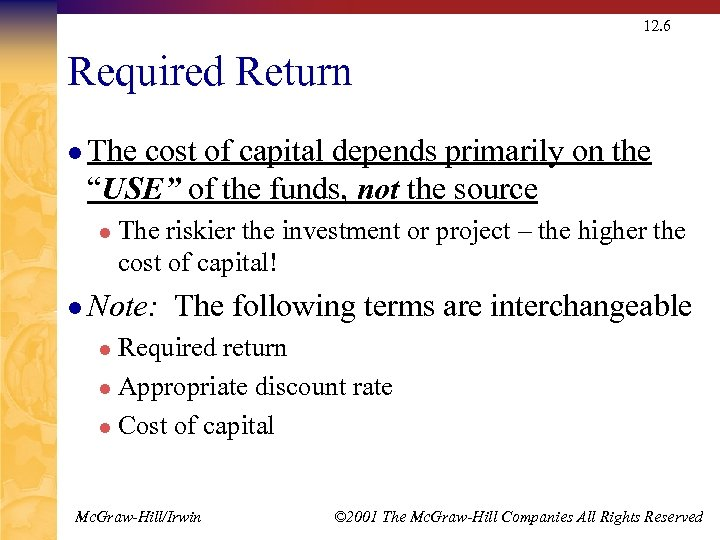12. 6 Required Return l The cost of capital depends primarily on the “USE” of the funds, not the source l The riskier the investment or project – the higher the cost of capital! l Note: The following terms are interchangeable Required return l Appropriate discount rate l Cost of capital l Mc. Graw-Hill/Irwin © 2001 The Mc. Graw-Hill Companies All Rights Reserved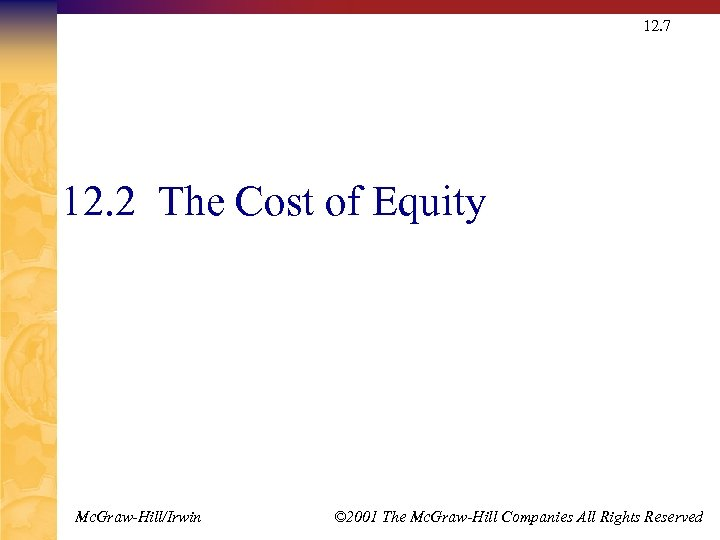12. 7 12. 2 The Cost of Equity Mc. Graw-Hill/Irwin © 2001 The Mc. Graw-Hill Companies All Rights Reserved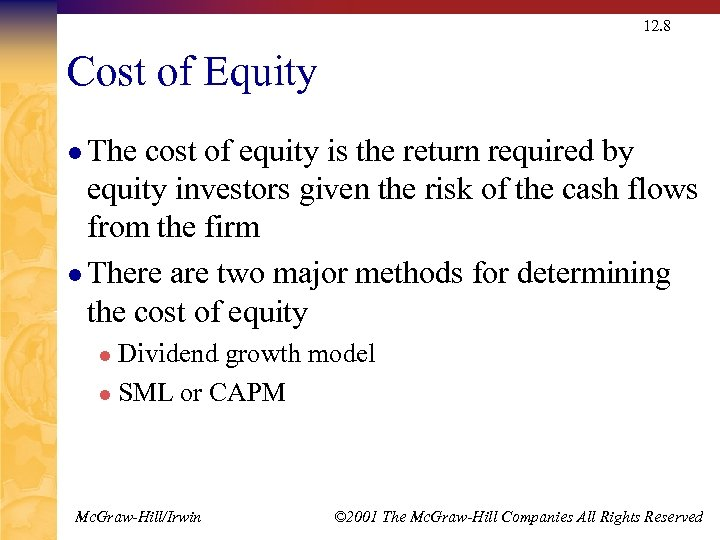12. 8 Cost of Equity l The cost of equity is the return required by equity investors given the risk of the cash flows from the firm l There are two major methods for determining the cost of equity Dividend growth model l SML or CAPM l Mc. Graw-Hill/Irwin © 2001 The Mc. Graw-Hill Companies All Rights Reserved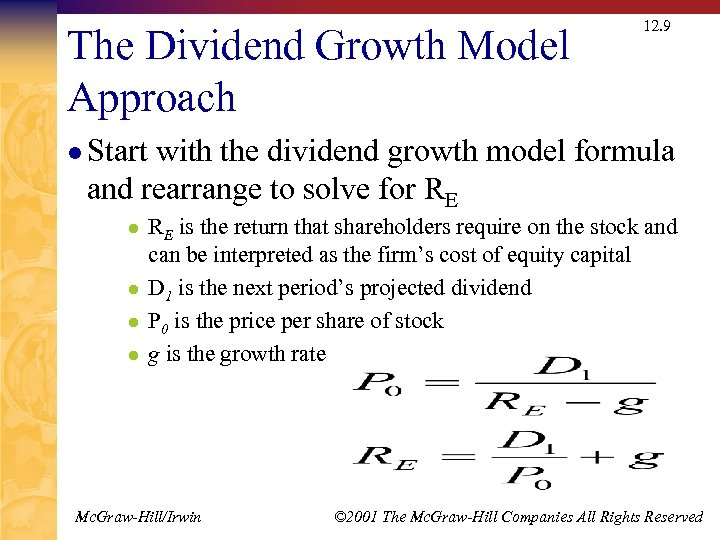The Dividend Growth Model Approach 12. 9 l Start with the dividend growth model formula and rearrange to solve for RE l l RE is the return that shareholders require on the stock and can be interpreted as the firm’s cost of equity capital D 1 is the next period’s projected dividend P 0 is the price per share of stock g is the growth rate Mc. Graw-Hill/Irwin © 2001 The Mc. Graw-Hill Companies All Rights Reserved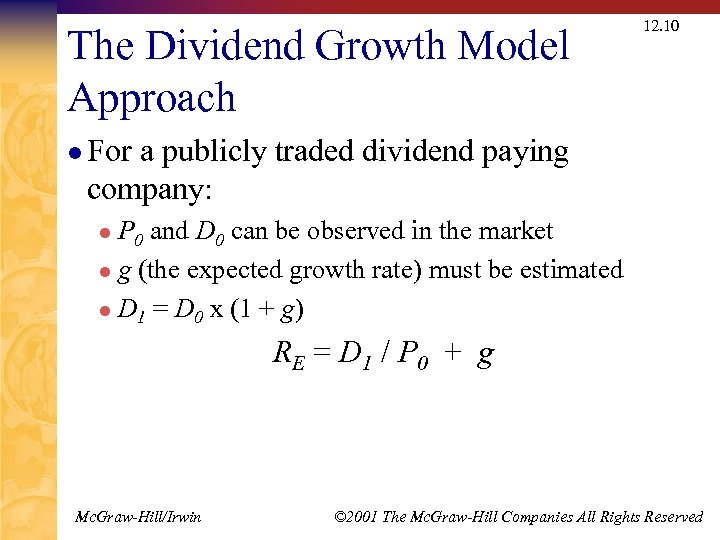The Dividend Growth Model Approach 12. 10 l For a publicly traded dividend paying company: P 0 and D 0 can be observed in the market l g (the expected growth rate) must be estimated l D 1 = D 0 x (1 + g) l R E = D 1 / P 0 + g Mc. Graw-Hill/Irwin © 2001 The Mc. Graw-Hill Companies All Rights Reserved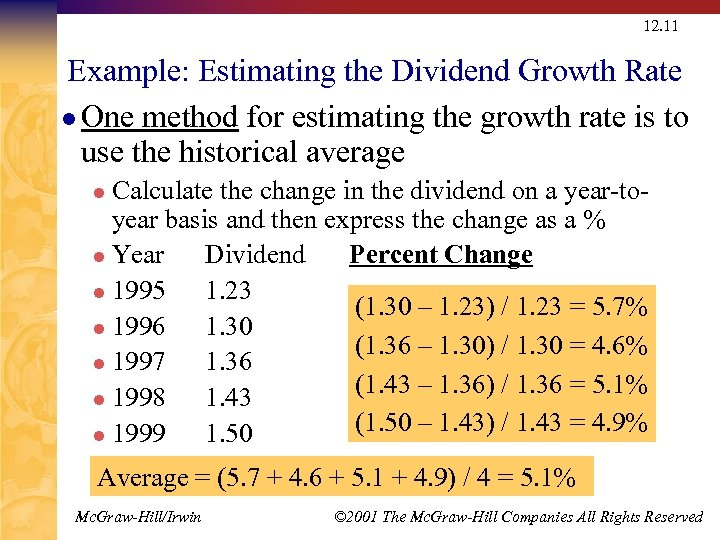12. 11 Example: Estimating the Dividend Growth Rate l One method for estimating the growth rate is to use the historical average Calculate the change in the dividend on a year-toyear basis and then express the change as a % l Year Dividend Percent Change l 1995 1. 23 (1. 30 – 1. 23) / 1. 23 = 5. 7% l 1996 1. 30 (1. 36 – 1. 30) / 1. 30 = 4. 6% l 1997 1. 36 (1. 43 – 1. 36) / 1. 36 = 5. 1% l 1998 1. 43 (1. 50 – 1. 43) / 1. 43 = 4. 9% l 1999 1. 50 l Average = (5. 7 + 4. 6 + 5. 1 + 4. 9) / 4 = 5. 1% Mc. Graw-Hill/Irwin © 2001 The Mc. Graw-Hill Companies All Rights Reserved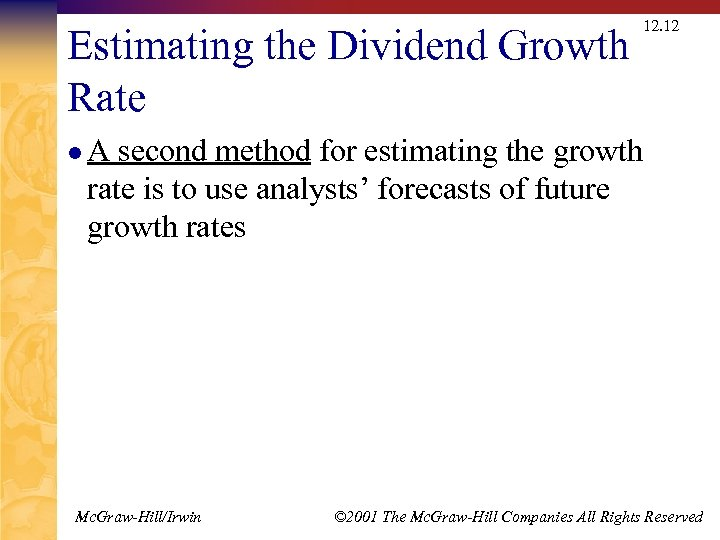Estimating the Dividend Growth Rate 12. 12 l. A second method for estimating the growth rate is to use analysts’ forecasts of future growth rates Mc. Graw-Hill/Irwin © 2001 The Mc. Graw-Hill Companies All Rights Reserved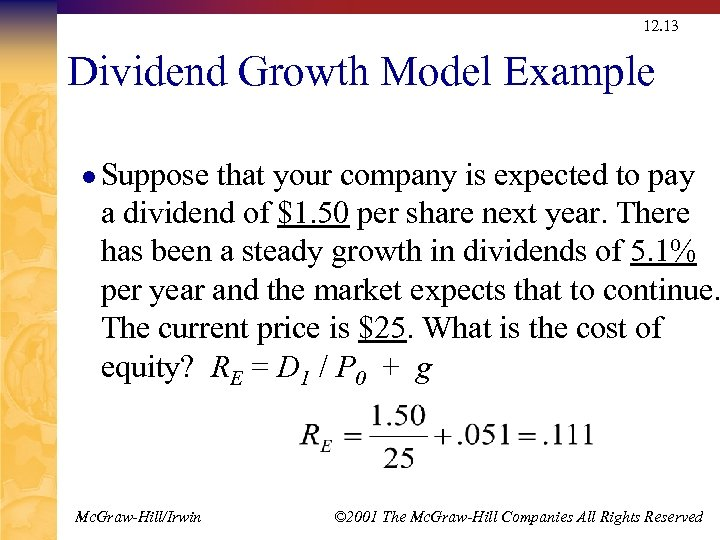12. 13 Dividend Growth Model Example l Suppose that your company is expected to pay a dividend of \$1. 50 per share next year. There has been a steady growth in dividends of 5. 1% per year and the market expects that to continue. The current price is \$25. What is the cost of equity? RE = D 1 / P 0 + g Mc. Graw-Hill/Irwin © 2001 The Mc. Graw-Hill Companies All Rights Reserved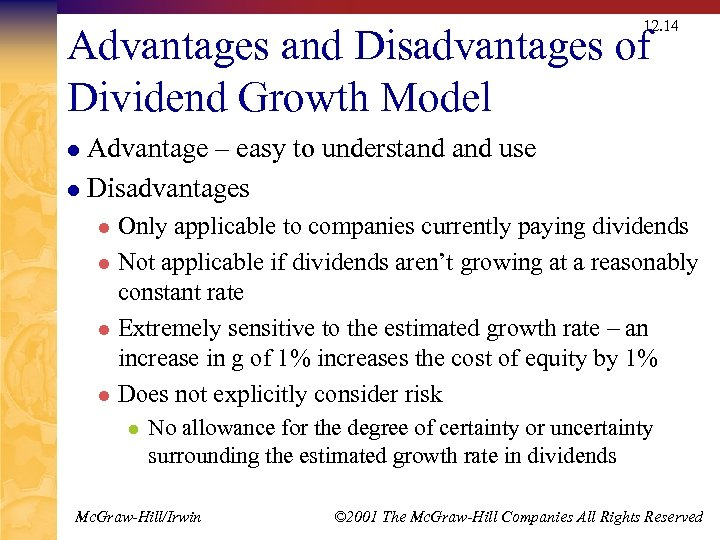12. 14 Advantages and Disadvantages of Dividend Growth Model Advantage – easy to understand use l Disadvantages l Only applicable to companies currently paying dividends l Not applicable if dividends aren’t growing at a reasonably constant rate l Extremely sensitive to the estimated growth rate – an increase in g of 1% increases the cost of equity by 1% l Does not explicitly consider risk l l No allowance for the degree of certainty or uncertainty surrounding the estimated growth rate in dividends Mc. Graw-Hill/Irwin © 2001 The Mc. Graw-Hill Companies All Rights Reserved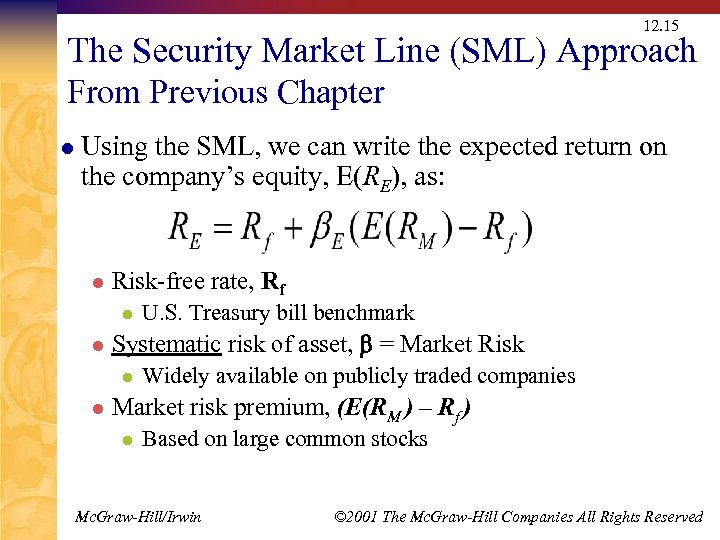12. 15 The Security Market Line (SML) Approach From Previous Chapter l Using the SML, we can write the expected return on the company’s equity, E(RE), as: l Risk-free rate, Rf l l Systematic risk of asset, = Market Risk l l U. S. Treasury bill benchmark Widely available on publicly traded companies Market risk premium, (E(RM ) – Rf ) l Based on large common stocks Mc. Graw-Hill/Irwin © 2001 The Mc. Graw-Hill Companies All Rights Reserved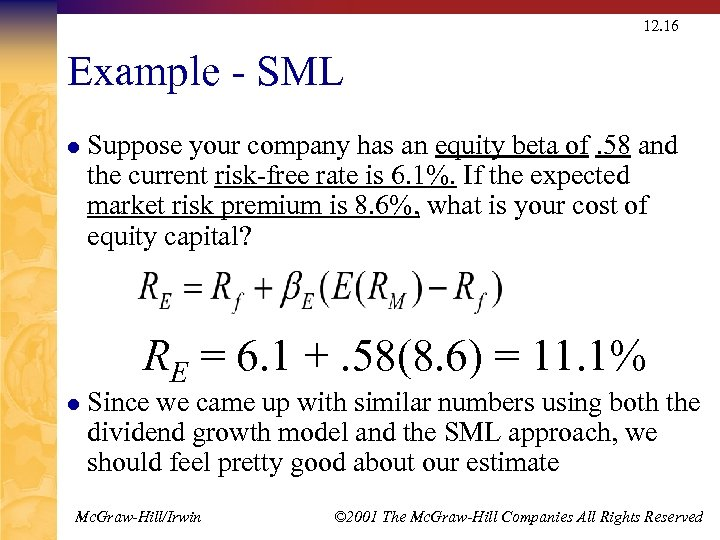12. 16 Example - SML l Suppose your company has an equity beta of. 58 and the current risk-free rate is 6. 1%. If the expected market risk premium is 8. 6%, what is your cost of equity capital? RE = 6. 1 +. 58(8. 6) = 11. 1% l Since we came up with similar numbers using both the dividend growth model and the SML approach, we should feel pretty good about our estimate Mc. Graw-Hill/Irwin © 2001 The Mc. Graw-Hill Companies All Rights Reserved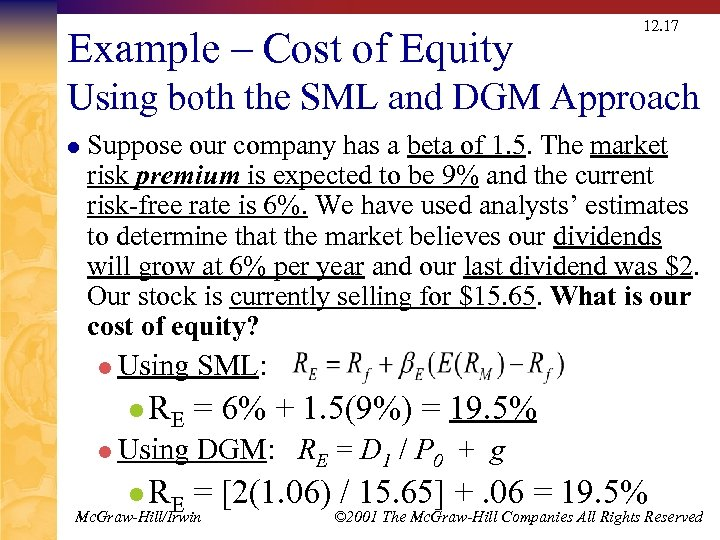Example – Cost of Equity 12. 17 Using both the SML and DGM Approach l Suppose our company has a beta of 1. 5. The market risk premium is expected to be 9% and the current risk-free rate is 6%. We have used analysts’ estimates to determine that the market believes our dividends will grow at 6% per year and our last dividend was \$2. Our stock is currently selling for \$15. 65. What is our cost of equity? l Using l RE SML: = 6% + 1. 5(9%) = 19. 5% DGM: RE = D 1 / P 0 + g = [2(1. 06) / 15. 65] +. 06 = 19. 5% Mc. Graw-Hill/Irwin © 2001 The Mc. Graw-Hill Companies All Rights Reserved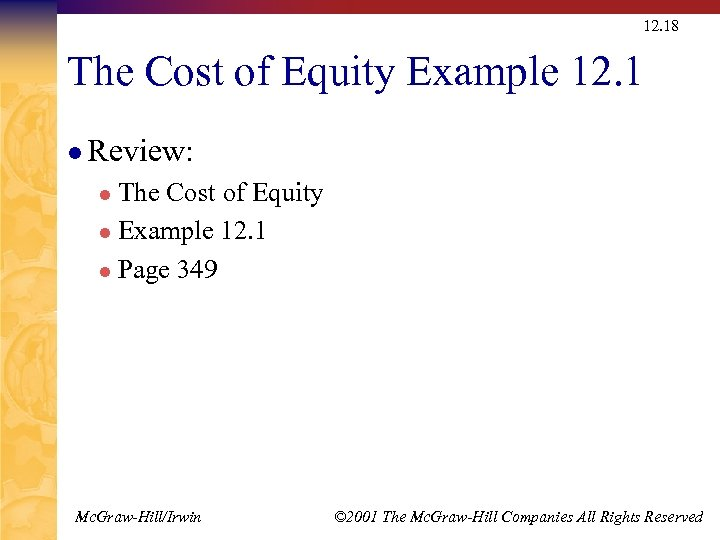12. 18 The Cost of Equity Example 12. 1 l Review: The Cost of Equity l Example 12. 1 l Page 349 l Mc. Graw-Hill/Irwin © 2001 The Mc. Graw-Hill Companies All Rights Reserved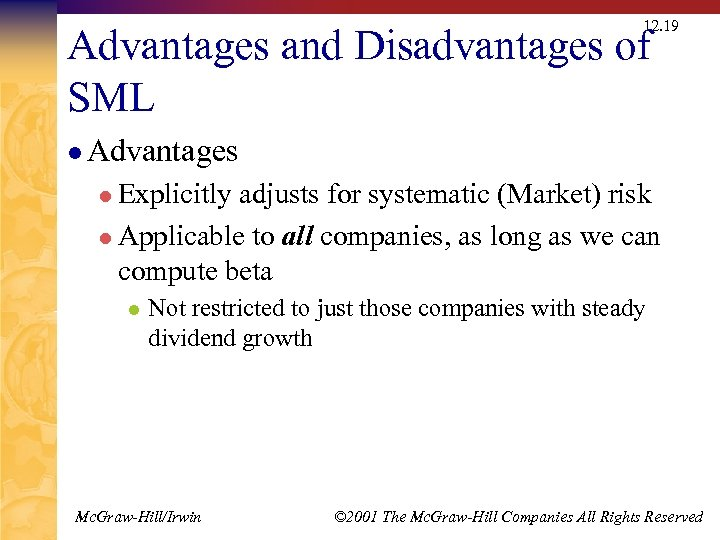12. 19 Advantages and Disadvantages of SML l Advantages l Explicitly adjusts for systematic (Market) risk l Applicable to all companies, as long as we can compute beta l Not restricted to just those companies with steady dividend growth Mc. Graw-Hill/Irwin © 2001 The Mc. Graw-Hill Companies All Rights Reserved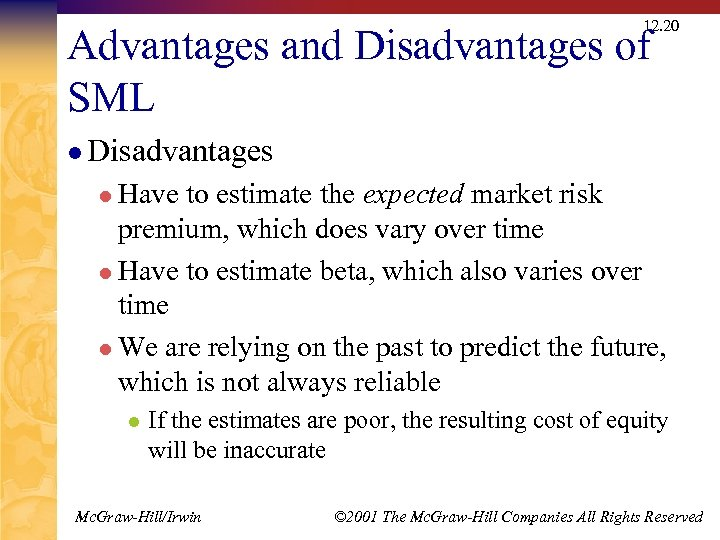12. 20 Advantages and Disadvantages of SML l Disadvantages l Have to estimate the expected market risk premium, which does vary over time l Have to estimate beta, which also varies over time l We are relying on the past to predict the future, which is not always reliable l If the estimates are poor, the resulting cost of equity will be inaccurate Mc. Graw-Hill/Irwin © 2001 The Mc. Graw-Hill Companies All Rights Reserved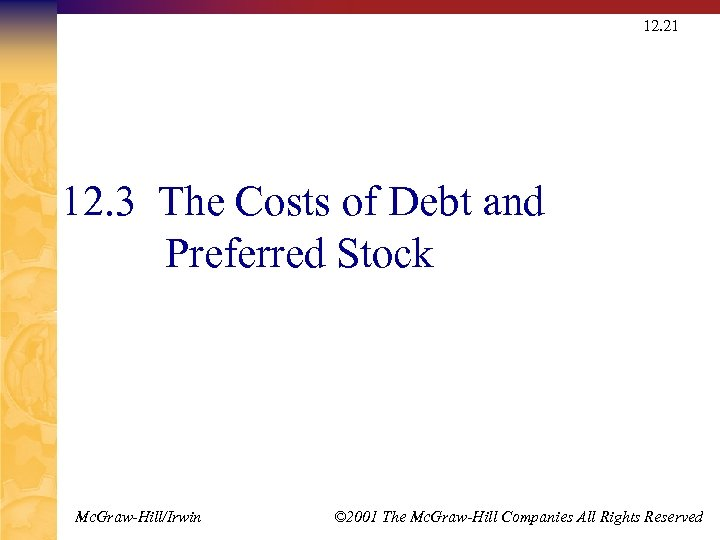12. 21 12. 3 The Costs of Debt and Preferred Stock Mc. Graw-Hill/Irwin © 2001 The Mc. Graw-Hill Companies All Rights Reserved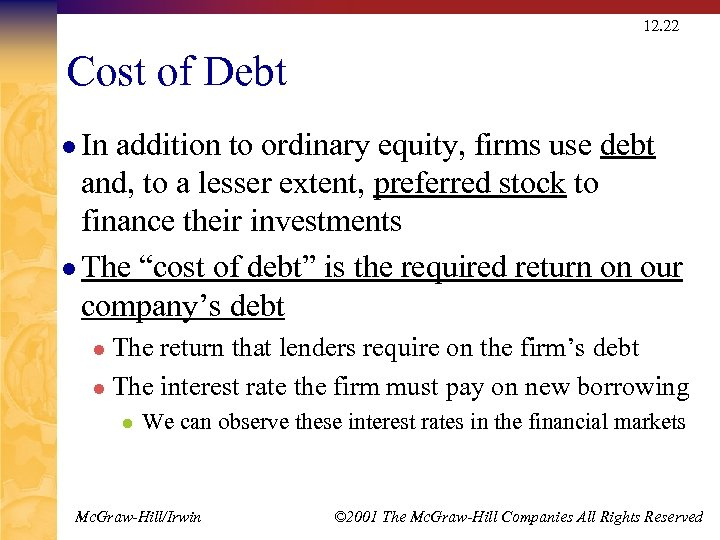12. 22 Cost of Debt l In addition to ordinary equity, firms use debt and, to a lesser extent, preferred stock to finance their investments l The “cost of debt” is the required return on our company’s debt The return that lenders require on the firm’s debt l The interest rate the firm must pay on new borrowing l l We can observe these interest rates in the financial markets Mc. Graw-Hill/Irwin © 2001 The Mc. Graw-Hill Companies All Rights Reserved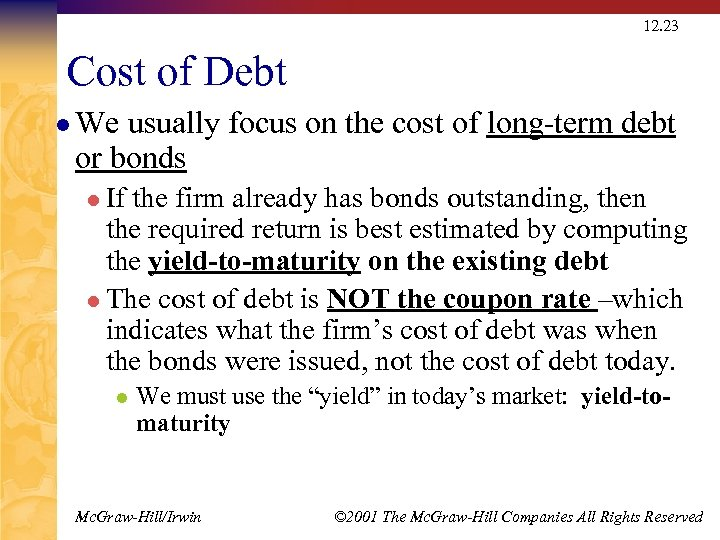12. 23 Cost of Debt l We usually focus on the cost of long-term debt or bonds l If the firm already has bonds outstanding, then the required return is best estimated by computing the yield-to-maturity on the existing debt l The cost of debt is NOT the coupon rate –which indicates what the firm’s cost of debt was when the bonds were issued, not the cost of debt today. l We must use the “yield” in today’s market: yield-tomaturity Mc. Graw-Hill/Irwin © 2001 The Mc. Graw-Hill Companies All Rights Reserved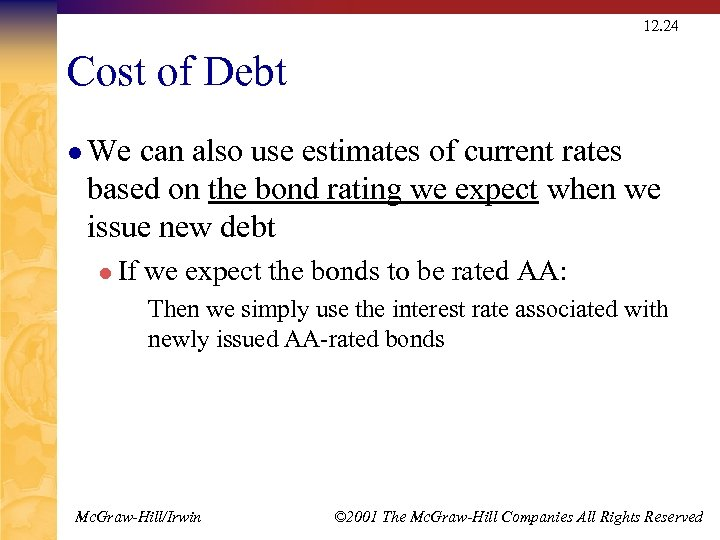12. 24 Cost of Debt l We can also use estimates of current rates based on the bond rating we expect when we issue new debt l If we expect the bonds to be rated AA: Then we simply use the interest rate associated with newly issued AA-rated bonds Mc. Graw-Hill/Irwin © 2001 The Mc. Graw-Hill Companies All Rights Reserved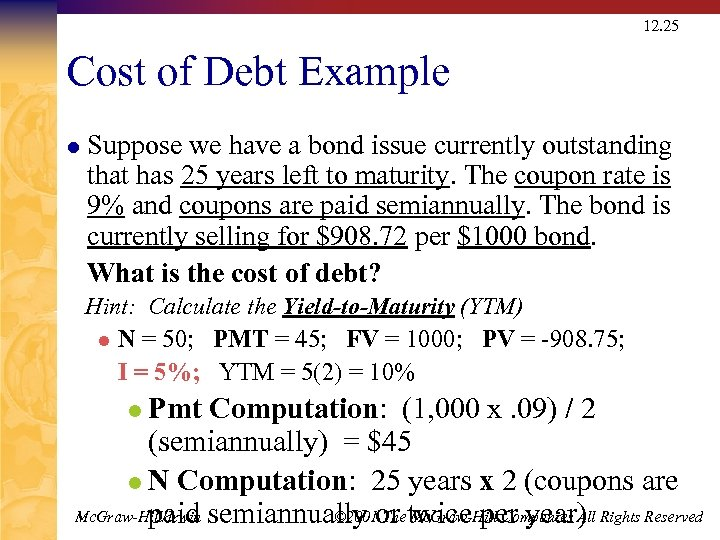12. 25 Cost of Debt Example l Suppose we have a bond issue currently outstanding that has 25 years left to maturity. The coupon rate is 9% and coupons are paid semiannually. The bond is currently selling for \$908. 72 per \$1000 bond. What is the cost of debt? Hint: Calculate the Yield-to-Maturity (YTM) l N = 50; PMT = 45; FV = 1000; PV = -908. 75; I = 5%; YTM = 5(2) = 10% l Pmt Computation: (1, 000 x. 09) / 2 (semiannually) = \$45 l N Computation: 25 years x 2 (coupons are Mc. Graw-Hill/Irwin semiannually or twice per year) Rights Reserved © 2001 The Mc. Graw-Hill Companies All paid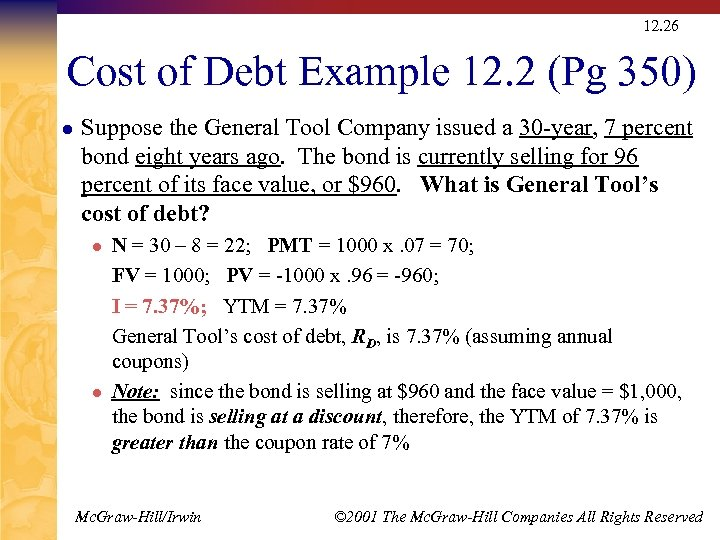12. 26 Cost of Debt Example 12. 2 (Pg 350) l Suppose the General Tool Company issued a 30 -year, 7 percent bond eight years ago. The bond is currently selling for 96 percent of its face value, or \$960. What is General Tool’s cost of debt? l l N = 30 – 8 = 22; PMT = 1000 x. 07 = 70; FV = 1000; PV = -1000 x. 96 = -960; I = 7. 37%; YTM = 7. 37% General Tool’s cost of debt, RD, is 7. 37% (assuming annual coupons) Note: since the bond is selling at \$960 and the face value = \$1, 000, the bond is selling at a discount, therefore, the YTM of 7. 37% is greater than the coupon rate of 7% Mc. Graw-Hill/Irwin © 2001 The Mc. Graw-Hill Companies All Rights Reserved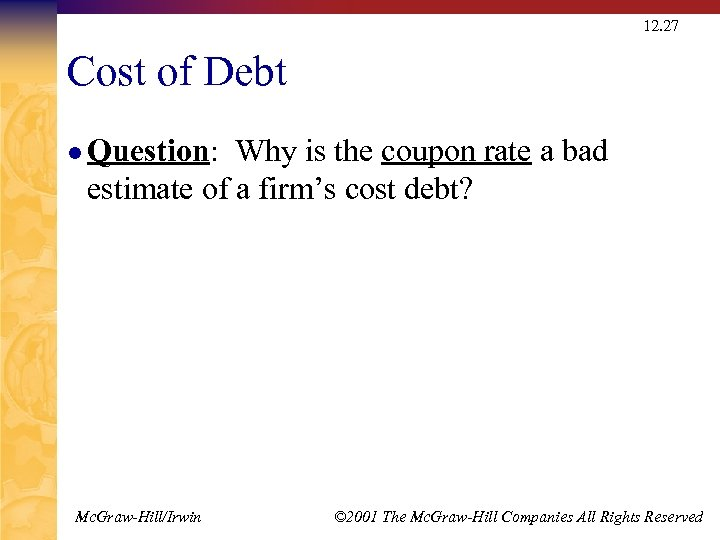12. 27 Cost of Debt l Question: Why is the coupon rate a bad estimate of a firm’s cost debt? Mc. Graw-Hill/Irwin © 2001 The Mc. Graw-Hill Companies All Rights Reserved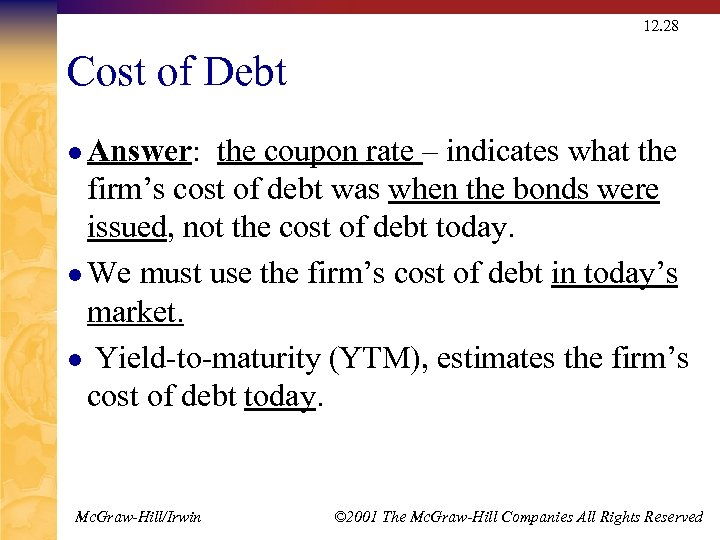12. 28 Cost of Debt l Answer: the coupon rate – indicates what the firm’s cost of debt was when the bonds were issued, not the cost of debt today. l We must use the firm’s cost of debt in today’s market. l Yield-to-maturity (YTM), estimates the firm’s cost of debt today. Mc. Graw-Hill/Irwin © 2001 The Mc. Graw-Hill Companies All Rights Reserved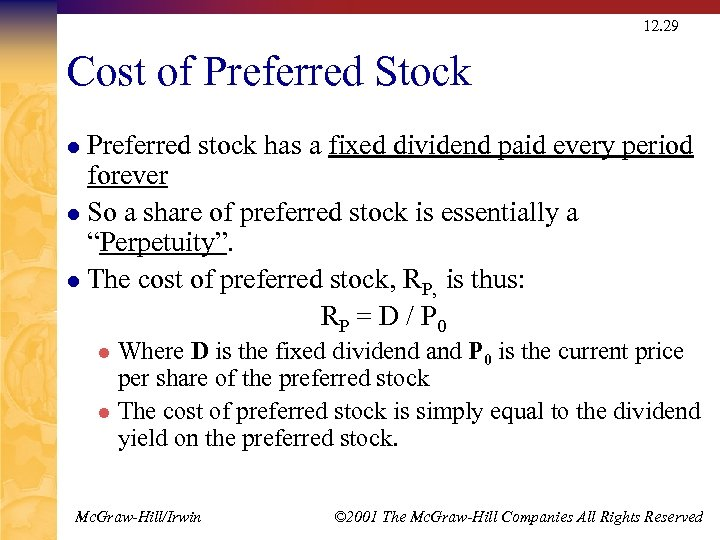12. 29 Cost of Preferred Stock Preferred stock has a fixed dividend paid every period forever l So a share of preferred stock is essentially a “Perpetuity”. l The cost of preferred stock, RP, is thus: RP = D / P 0 l Where D is the fixed dividend and P 0 is the current price per share of the preferred stock l The cost of preferred stock is simply equal to the dividend yield on the preferred stock. l Mc. Graw-Hill/Irwin © 2001 The Mc. Graw-Hill Companies All Rights Reserved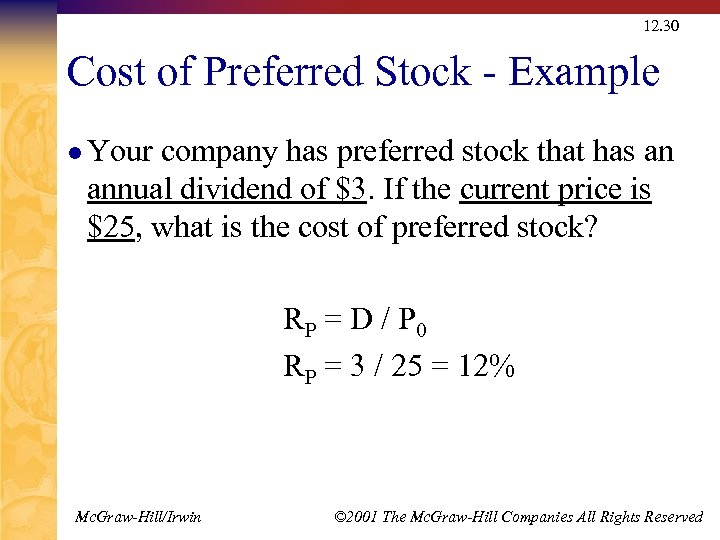12. 30 Cost of Preferred Stock - Example l Your company has preferred stock that has an annual dividend of \$3. If the current price is \$25, what is the cost of preferred stock? RP = D / P 0 RP = 3 / 25 = 12% Mc. Graw-Hill/Irwin © 2001 The Mc. Graw-Hill Companies All Rights Reserved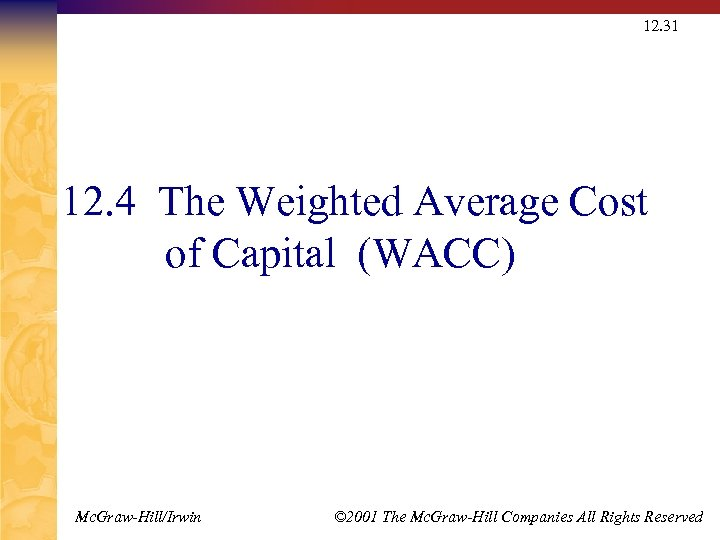12. 31 12. 4 The Weighted Average Cost of Capital (WACC) Mc. Graw-Hill/Irwin © 2001 The Mc. Graw-Hill Companies All Rights Reserved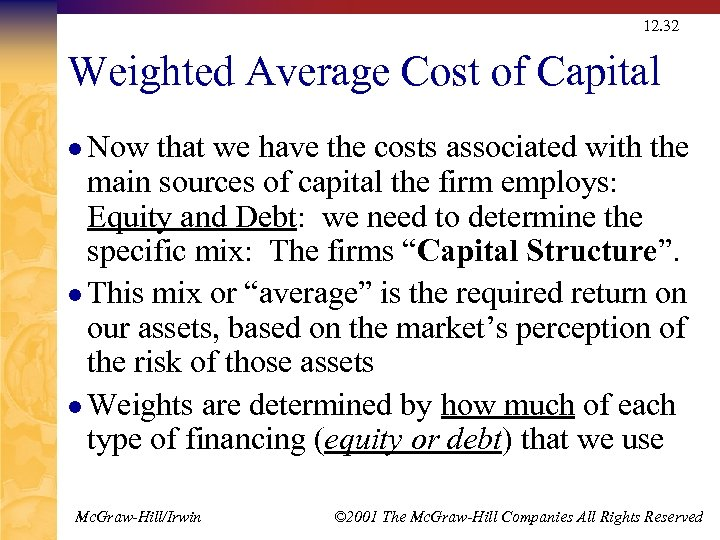12. 32 Weighted Average Cost of Capital l Now that we have the costs associated with the main sources of capital the firm employs: Equity and Debt: we need to determine the specific mix: The firms “Capital Structure”. l This mix or “average” is the required return on our assets, based on the market’s perception of the risk of those assets l Weights are determined by how much of each type of financing (equity or debt) that we use Mc. Graw-Hill/Irwin © 2001 The Mc. Graw-Hill Companies All Rights Reserved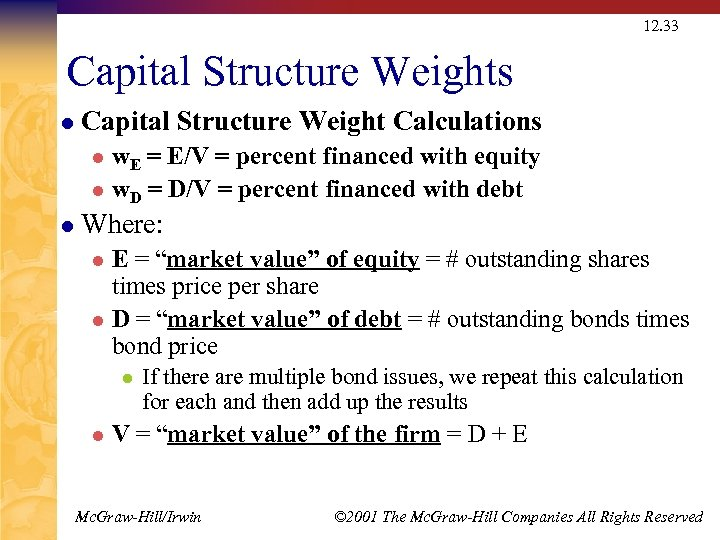12. 33 Capital Structure Weights l Capital Structure Weight Calculations w. E = E/V = percent financed with equity l w. D = D/V = percent financed with debt l l Where: E = “market value” of equity = # outstanding shares times price per share l D = “market value” of debt = # outstanding bonds times bond price l l l If there are multiple bond issues, we repeat this calculation for each and then add up the results V = “market value” of the firm = D + E Mc. Graw-Hill/Irwin © 2001 The Mc. Graw-Hill Companies All Rights Reserved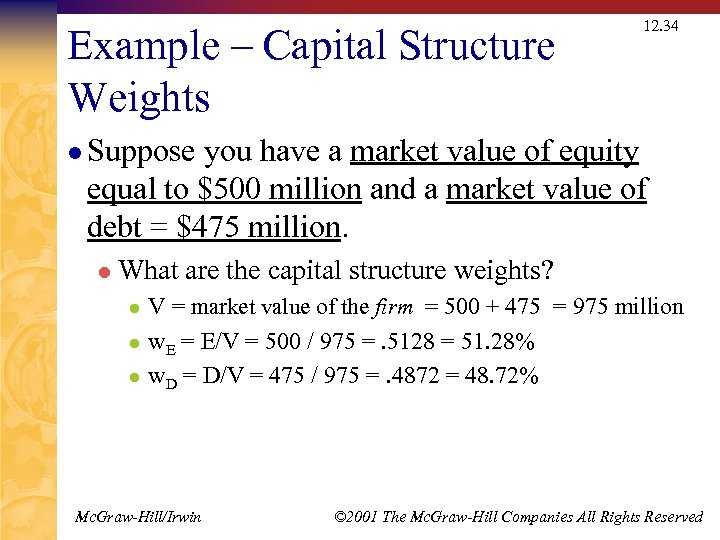Example – Capital Structure Weights 12. 34 l Suppose you have a market value of equity equal to \$500 million and a market value of debt = \$475 million. l What are the capital structure weights? l l l V = market value of the firm = 500 + 475 = 975 million w. E = E/V = 500 / 975 =. 5128 = 51. 28% w. D = D/V = 475 / 975 =. 4872 = 48. 72% Mc. Graw-Hill/Irwin © 2001 The Mc. Graw-Hill Companies All Rights Reserved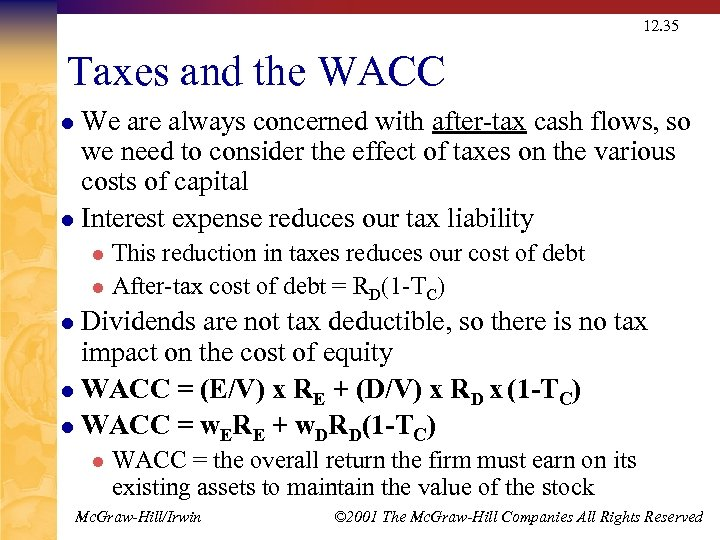12. 35 Taxes and the WACC We are always concerned with after-tax cash flows, so we need to consider the effect of taxes on the various costs of capital l Interest expense reduces our tax liability l This reduction in taxes reduces our cost of debt l After-tax cost of debt = RD(1 -TC) l Dividends are not tax deductible, so there is no tax impact on the cost of equity l WACC = (E/V) x RE + (D/V) x RD x (1 -TC) l WACC = w. ERE + w. DRD(1 -TC) l l WACC = the overall return the firm must earn on its existing assets to maintain the value of the stock Mc. Graw-Hill/Irwin © 2001 The Mc. Graw-Hill Companies All Rights Reserved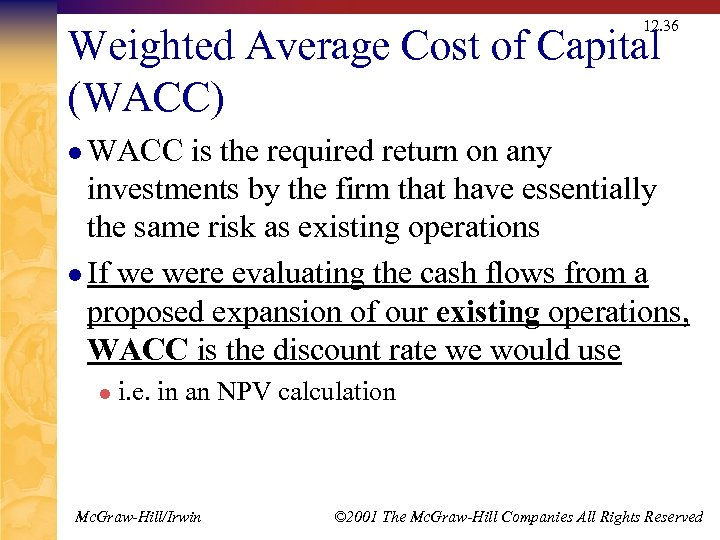12. 36 Weighted Average Cost of Capital (WACC) l WACC is the required return on any investments by the firm that have essentially the same risk as existing operations l If we were evaluating the cash flows from a proposed expansion of our existing operations, WACC is the discount rate we would use l i. e. in an NPV calculation Mc. Graw-Hill/Irwin © 2001 The Mc. Graw-Hill Companies All Rights Reserved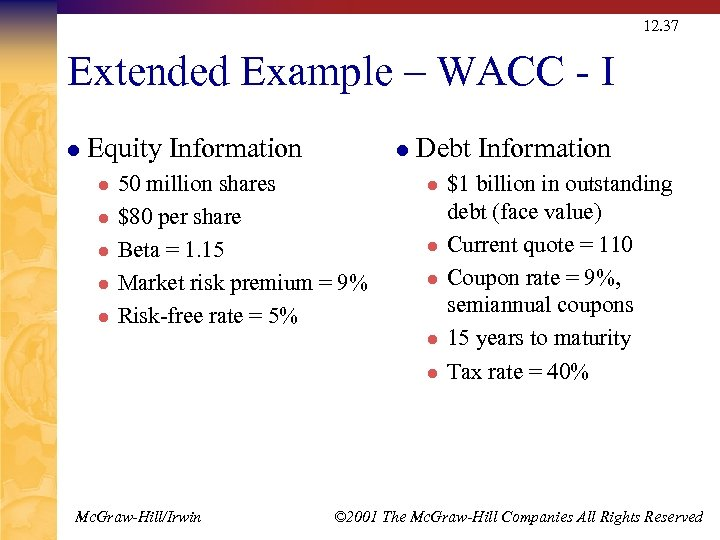12. 37 Extended Example – WACC - I l Equity Information l l l 50 million shares \$80 per share Beta = 1. 15 Market risk premium = 9% Risk-free rate = 5% Debt Information l l l Mc. Graw-Hill/Irwin \$1 billion in outstanding debt (face value) Current quote = 110 Coupon rate = 9%, semiannual coupons 15 years to maturity Tax rate = 40% © 2001 The Mc. Graw-Hill Companies All Rights Reserved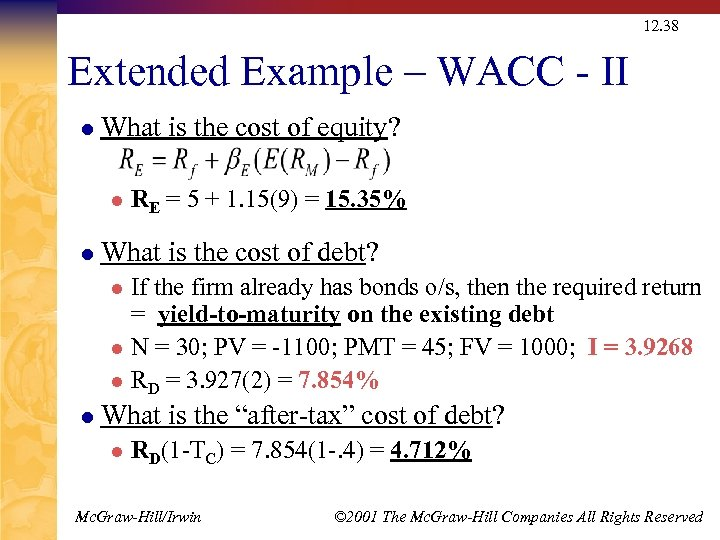12. 38 Extended Example – WACC - II l What is the cost of equity? l l RE = 5 + 1. 15(9) = 15. 35% What is the cost of debt? If the firm already has bonds o/s, then the required return = yield-to-maturity on the existing debt l N = 30; PV = -1100; PMT = 45; FV = 1000; I = 3. 9268 l RD = 3. 927(2) = 7. 854% l l What is the “after-tax” cost of debt? l RD(1 -TC) = 7. 854(1 -. 4) = 4. 712% Mc. Graw-Hill/Irwin © 2001 The Mc. Graw-Hill Companies All Rights Reserved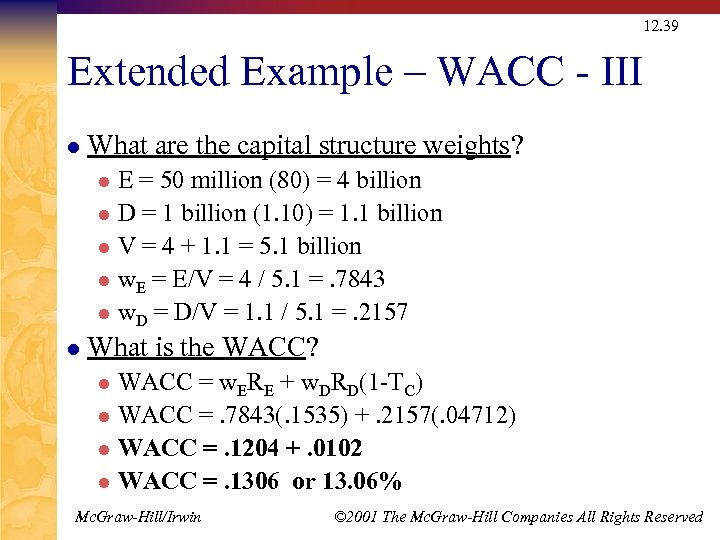12. 39 Extended Example – WACC - III l What are the capital structure weights? E = 50 million (80) = 4 billion l D = 1 billion (1. 10) = 1. 1 billion l V = 4 + 1. 1 = 5. 1 billion l w. E = E/V = 4 / 5. 1 =. 7843 l w. D = D/V = 1. 1 / 5. 1 =. 2157 l l What is the WACC? WACC = w. ERE + w. DRD(1 -TC) l WACC =. 7843(. 1535) +. 2157(. 04712) l WACC =. 1204 +. 0102 l WACC =. 1306 or 13. 06% l Mc. Graw-Hill/Irwin © 2001 The Mc. Graw-Hill Companies All Rights Reserved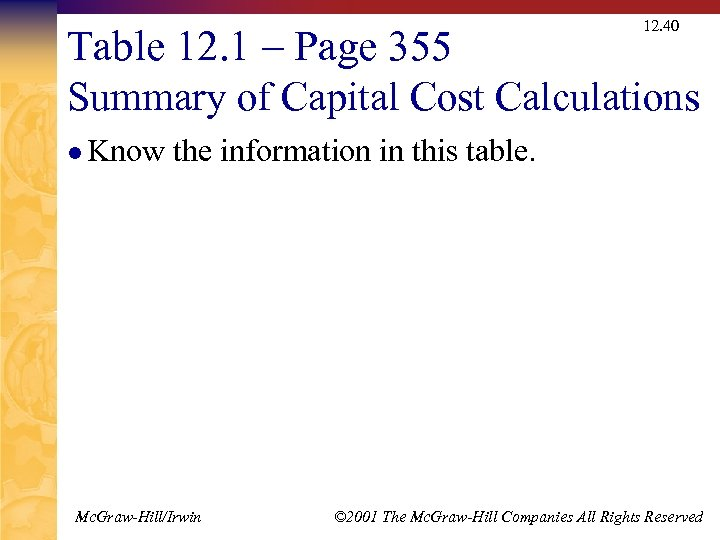12. 40 Table 12. 1 – Page 355 Summary of Capital Cost Calculations l Know the information in this table. Mc. Graw-Hill/Irwin © 2001 The Mc. Graw-Hill Companies All Rights Reserved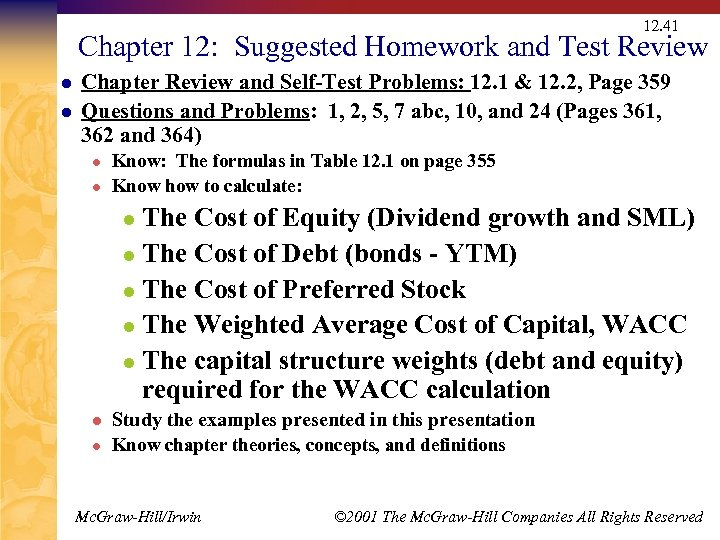12. 41 Chapter 12: Suggested Homework and Test Review l l Chapter Review and Self-Test Problems: 12. 1 & 12. 2, Page 359 Questions and Problems: 1, 2, 5, 7 abc, 10, and 24 (Pages 361, 362 and 364) l l Know: The formulas in Table 12. 1 on page 355 Know how to calculate: The Cost of Equity (Dividend growth and SML) l The Cost of Debt (bonds - YTM) l The Cost of Preferred Stock l The Weighted Average Cost of Capital, WACC l The capital structure weights (debt and equity) required for the WACC calculation l l Study the examples presented in this presentation l Know chapter theories, concepts, and definitions Mc. Graw-Hill/Irwin © 2001 The Mc. Graw-Hill Companies All Rights Reserved